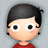#### 学习笔记TF049:TensorFlow 模型存储加载、队列线程、加载数据、自定义操作

``````#加载数据
trX,trY,teX,teY = mnist.train.images,mnist.train.labels,mnist.test.images,mnist.test.labels
X = tf.placeholder("float",[None,784])
Y = tf.placeholder("float",[None,10])
#初始化权重参数
w_h = init_weights([784,625])
w_h2 = init_weights([625,625])
w_o = int_weights([625,10])

#定义权重函数
def init_weights(shape):
return tf.Variable(tf.random_normal(shape,stddev=0.01))

#定义模型
def model(X,w_h,w_h2,w_o,p_keep_input,p_keep_hidden):
#第一个全连接层
X = tf.nn.dropout(X,p_keep_input)
h = tf.nn.relu(tf.matmul(X,w_h))

h = tf.nn.dropout(h,p_keep,hidden)
#第二个全连接层
h2 = tf.nn.relu(tf.matmul(h,w_h2))
h2 = tf.nn.dropout(h2,p_keep_hidden)

return tf.matmul(h2,w_o)#输出预测值

#定义损失函数
cost = tf.reduce_mean(tf.nn.softmax_cross_entropy_with_logits(py_x,Y))
train_op = tf.train.RMSPropOptimizer(0.001,0.9).minimize(cost)
predict_op = tf.argmax(py_x,1)
``````

``````#定义存储路径
ckpt_dir = "./ckpt_dir"
if not os.path.exists(ckpt_dir):
os.makedirs(ckpt_dir)
``````

``````#计数器变量，设置它的trainable=False，不需要被训练
global_step = tf.Variable(0,name='global_step',trainable=False)
``````

``````#声明完所有变量，调tf.train.Saver
saver = tf.train.Saver()
#之后变量不被存储
non_storable_variable = tf.Variable(777)
``````

``````with tf.Session() as sess:
tf.initialize_all_variables().run()
start = global_step.eval() #得到global_stepp初始值
print("Start from:",start)
for i in range(start,100):
#128 batch_size
for start,end in zip(range(0,len(trX),128),range(128,len(trX)+1,128)):
sess.run(train_op,feed_dict={X:trX[start:end],Y:trY[start:end],p_keep_input:0.8, p_keep_hidden:0.5})
global_step.assign(i).eval() #更新计数器
saver.save(sess,ckpt_dir+"/model.ckpt",global_step=global_step) #存储模型
``````

``````with tf.Session() as sess:
tf.initialize_all_variables().run()
ckpt = tf.train.get_checkpoint_state(ckpt_dir)
if ckpt and ckpt.model_checkpoint_path:
print(ckpt.model_checkpoint_path)
saver.restore(sess,ckpt.model_checkpoint_path) #加载所有参数
``````

``````v = tf.Variable(0,name='my_variable')
sess = tf.Session()
tf.train.write_graph(sess.graph_def,'/tmp/tfmodel','train.pbtxt')
``````

``````with tf.Session() as _sess:
with grile.FastGFile("/tmp/tfmodel/train.pbtxt",'rb') as f:
graph_def = tf.GraphDef()
_sess.graph.as_default()
tf.import_graph_def(graph_def,name='tfgraph')
``````

``````import tensorflow as tf
#创建先入先出队列，初始化队列插入0.1、0.2、0.3数字
q = tf.FIFOQueue(3,"float")
init = q.enqueue_many(([0.1,0.2,0.3]))
#定义出队、+1、入队操作
x = q.dequeue()
y = x +1
q_inc = q.enqueue([y])
``````

``````with tf.Session() as sess:
sess.run(init)
quelen = sess.run(q.size())
for i in range(2):
sess.run(q_inc) #执行2次操作，队列值变0.3,1.1,1.2
quelen = sess.run(q.size())
for i in range(quelen):
print(sess.run(q.dequeue())) #输出队列值
``````

RandomShuffleQueue，创建随机队列。出队列，以随机顺序产生元素。训练图像样本，CNN网络结构，无序读入训练样本，每次随机产生一个训练样本。 异步计算时非常重要。TensorFlow会话支持多线程，在主线程训练操作，RandomShuffleQueue作训练输入，开多个线程准备训练样本。样本压入队列，主线程从队列每次取出mini-batch样本训练。 创建随机队列，最大长度10,出队后最小长度2:

``````q = tf.RandomShuffleQueue(capacity=10,min_after_dequeue=2,dtype="float")
``````

``````sess = tf.Session()
for i in range(0,10): #10次入队
sess.run(q.enqueue(i))
for i in range(0,8): #8次出队
print(sess.run(q.dequeue()))
``````

``````run_iptions = tf.RunOptions(timeout_in_ms = 10000) #等待10秒
try:
sess.run(q.dequeue(),options=run_options)
print('out of range')
``````

``````q = tf.FIFOQueue(1000,"float")
counter = tf.Variable(0.0) #计数器
enqueue_op = q.enqueue(counter) #操作:计数器值加入队列
``````

``````qr = tf.train.QueueRunner(q,enqueue_ops=[increment_op,enqueue_op] * 1)
``````

``````#主线程
with tf.Session() as sess:
sess.run(tf.global_variables_initializer())
#主线程
for i in range(10):
print(sess.run(q.dequeue()))
``````

``````#主线程
sess = tf.Session()
sess.run(tf.global_variables_initializer())
#Coordinator:协调器，协调线程间关系可以视为一种信号量，用来做同步
coord = tf.train.Coordinator()
#启动入队线程，协调器是线程的参数
#主线程
for i in range(0,10):
print(sess.run(q.dequeue()))
coord.request_stop() #通知其他线程关闭
``````

``````coord.request_stop()
#主线程
for i in range(0,10):
print(sess.run(q.dequeue()))
tf.errors.OutOfRangeError捕捉错误：
coord.request_stop()
#主线程
for i in range(0,10):
try:
print(sess.run(q.dequeue()))
except tf.errors.OutOfRangeError:
break
``````

``````x1 = tf.constant([2,3,4])
x2 = tf.constant([4,0,1])
``````

``````import tensorflow as tf
#设计图
a1 = tf.placeholder(tf.int16)
a2 = tf.placeholder(tf.int16)
#用Python产生数据
li1 = [2,3,4]
li2 = [4,0,1]
#打开会话，数据填充后端
with tf.Session() as sess:
print sess.run(b,feed_dict={a1:li1,a2:li2})
``````